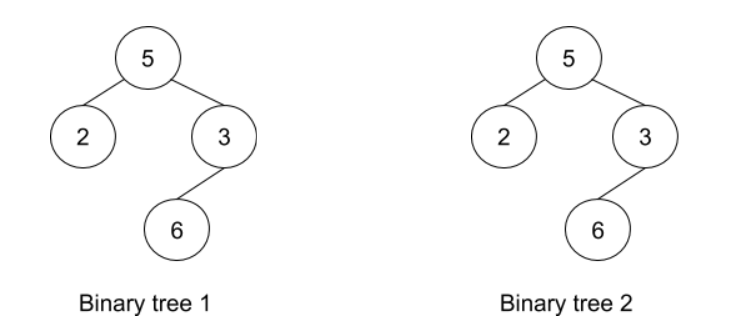# Check Identical Trees

Posted: 17 Oct, 2020
Difficulty: Moderate

## PROBLEM STATEMENT

#### Below is the example and explanation of Identical trees.

##### Example :
`````` For the trees given below:-
````````````The given trees are identical as:-
1. The number of nodes in both trees is the same.
2. The number of edges in both trees is the same.
3. The data for root for both the trees is the same i.e 5.
4. The data of root -> left (root’s left child) for both the trees is the same i.e 2.
5. The data of root -> right (root’s right child) for both the trees is the same i.e 3.
6. The data of root -> right -> left ( left child of root’s right child) for both the trees is the same i.e 6.
7. Nodes with data 2 and 6 are the leaf nodes for both the binary trees.
``````
##### Input format :
``````The first line of input contains an integer T denoting the number of queries or test cases.

The first line of every test case contains elements in the level order form for the first binary tree. The line consists of values of nodes separated by a single space. In case a node is null, we take -1 on its place.

The second line of every test case contains elements in the level order form for the second tree. The line consists of values of nodes separated by a single space. In case a node is null, we take -1 on its place.

For example, the level order input for the tree depicted in the below image would be :
````````````1
2 3
4 -1 5 6
-1 7 -1 -1 -1 -1
-1 -1
``````

#### Explanation :

``````Level 1 :
The root node of the tree is 1

Level 2 :
Left child of 1 = 2
Right child of 1 = 3

Level 3 :
Left child of 2 = 4
Right child of 2 = null (-1)
Left child of 3 = 5
Right child of 3 = 6

Level 4 :
Left child of 4 = null (-1)
Right child of 4 = 7
Left child of 5 = null (-1)
Right child of 5 = null (-1)
Left child of 6 = null (-1)
Right child of 6 = null (-1)

Level 5 :
Left child of 7 = null (-1)
Right child of 7 = null (-1)

The first not-null node (of the previous level) is treated as the parent of the first two nodes of the current level. The second not-null node (of the previous level) is treated as the parent node for the next two nodes of the current level and so on.
The input ends when all nodes at the last level are null (-1).
``````
##### Note :
``````The above format was just to provide clarity on how the input is formed for a given tree.
The sequence will be put together in a single line separated by a single space. Hence, for the above-depicted tree, the input will be given as:

1 2 3 4 -1 5 6 -1 7 -1 -1 -1 -1 -1 -1
``````
##### Output format :
``````For each test case, print in a single line either “True” (if the two trees are identical) or “False” otherwise.

The output of each test case will be printed in a separate line.
``````
##### Note :
``````You do not need to print anything, it has already been taken care of. Just implement the given function.
``````
##### Constraints :
``````1 <= T <= 10
0 <= N <= 10^5
0 <= M <= 10^5
1 <= Node Data <= 10^9

Time limit: 1 sec
``````Approach 1

The idea here is that we will store the level order traversal for both the trees in two lists and as the level order traversal for identical trees must be the same so we will check whether both the lists are the same or not. So the steps will be as given below.

1. Maintain an array say levelOrderTree1 and store the level order traversal of Binary tree 1 taking NULL nodes as ‘-1’.
2. Maintain an array say levelOrderTree2 and store the level order traversal of Binary tree 2 taking NULL nodes as ‘-1’.
3. Check if the two arrays are identical or not. If any of the indexes from both arrays mismatch return false otherwise return true.